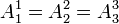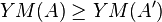## Is Terry wrong?

I am a great estimator of Terry Tao and a reader of his blog. Tao is a Fields medalist and one of the greatest living mathematicians. Relying on such a giant authority may give someone the feeling of being a kind of dwarf trying to be listened around. Anyhow I will try. Terry come out with an intervention in Wikipedia here claiming:

“It may be relevant to point out that one of the references cited in the disputed section  has a significant error in it, despite being published. Namely, in the proof of Theorem 1, the author is assuming that an extremum A for the Yang-Mills action for a special class of connections (namely those in which$A^1_1=A^2_2=A^3_3$ and all other components vanish) is necessarily an extremum for the Yang-Mills action for all other connections also, but this is not the case (just because$YM(A) \geq YM(A')$, for instance, for A’ of this special form, does not imply that$YM(A) \geq YM(A')$ for general A’). Since one needs to be an extremiser (or critical point) in the space of all connections in order to be a solution to the Yang-Mills equations, the mapping provided in Theorem 1 has not been shown to actually produce solutions to the Yang-Mills equation (and I suspect that if one actually checks the Yang-Mills equation for this mapping, that one will not in fact get such a solution). Terry (talk) 20:32, 28 February 2009 (UTC)”

This claim of mistake by my side contains a misinterpretation of the mapping theorem. If the theorem would claim that this is true for all connections, as Terry says, it would be istantaneously false. I cannot map a scalar field on all the Y-M connections (think of chaotic solutions). The theorem simply states that there exists a class of solutions of the quartic scalar field that are also solution for the Yang-Mills equations and this can be easily proved by substitution (check Smilga’s book) and Tao is proved istantaneously wrong. So now, what is the point? I have a class of Yang-Mills solutions that Tao is claiming are not. But whoever can check by herself that I am right. So, is Terry wrong?

### 13 Responses to Is Terry wrong?

1.Michael Dinsdale says:

I think you’ve misunderstood what Terry is getting at…

Whereas you can certainly find a set of solutions of a theory by substituting an ansatz into the *equations of motion*, you can’t do this with the action. The reason is that for a field configuration to be a solution the action must be stationary with respect to all infinitesimal variations of the fields, not just those which conform to your ansatz. But if you carry out your substitution and end up with your action written in terms of phi, and then derive an EOM from this, the action will only be stationary with respect to variations of phi. That means, in terms of the original variables, that the action will be invariant with respect to variations of A which still satisfy your ansatz. This is a necessary but not sufficient condition for the A you obtain from your phi to satisfy the YM EOM.

Another loose way of saying it would be that restricting to a set of solutions and finding a stationary point don’t commute.

(I hope that makes sense! What do you think?)

Anyway for this reason I think the proof you give in your paper is invalid. Also the result you get seems to be wrong for the reason Terry points out on the Wikipedia page (i.e. look at the YM eqn with a=1,v=2…)

Does Smilga really give the exact result you state? Could you quote the passage?

michael

•mfrasca says:

Dear Michael,

thank you for explaining this. I am not saying that Tao’s argument is untrue (I never heard wrong statements by him). The point is that for my proof I need a restrict class of integrable solutions and this class indeed exists and all the argument does hold. The point is, how one could change the demonstration of the theorem to get the proof straight? I can simply restate this theorem saying that a class of solutions exists that are commons to both theories and this is easily obtained by substitution. SO, I think that Terry’s criticism is just a mathematical subtlety that does not apply in this case.

For a full proof of existence see my post here https://marcofrasca.wordpress.com/2009/03/11/i-did-it-for-you/

I am somewhat shocked how one can lose elementary ways to verify a result. Indeed, Terry claims that such solutions could not exist! This is blatantly false.

Marco

2.unit says:

ciao Marco,
ti seguo con interesse e mi piace il tuo blog, ma qui a me sembra che Tao abbia ragione. Nel teorema 1 del tuo paper tu dimostri che l’azione di YM per *una certa classe di connessioni* è uguale all’azione del campo scalare con il potenziale quartico. Questo è chiaramente corretto. L’errore consiste nel passo successivo che metti in evidenza nelle due righe dopo la dimostrazione del teorema, dicendo che dato che le azioni sono uguali allora una soluzione del campo scalare è anche una soluzione delle equazioni di YM. Questo è falso, perchè potrebbe esserci un’altra connessione che non rientra nella classe che tu hai definito e che estremizza l’azione, e allora QUELLA connessione sarebbe soluzione delle equazioni di YM e non la corrispondente soluzione di campo scalare. Quando Tao parla di connessioni che non corrispondono, non parla di *soluzioni* che non corrispondono, ma di configurazioni della connessione sulla quale agisce il funzionale dell’azione che non sono “coperte” dalle corrispondenti configurazioni (non soluzioni) del campo scalare che tu fai variare alla ricerca dell’estremo. E in effetti ti mostra anche che quella che tu affermi essere una soluzione alle equazioni di YM in effetti non lo è.

Ciao!

3.Luboš Motl says:

Well, it depends on whether you have an independent proof that it is actually a solution.

If your only “proof” is that it extremizes the action within a limited class of configurations, those that satisfy additional conditions, then the “proof” is clearly false and it should be expected that the “solution” is actually not a solution and Terry is correct in the strongest possible sense. 😉

4.Ehab says:

Did you try emailing him ?

E.

5.mfrasca says:

Dear Lubos,

the independent proof is simply to avoid to use functionals and variational principles and working with equations of motion. In this case I am easily proved right. But I cannot always map such scalar field solutions and this is where Tao’s criticism applies correctly.

Dear Ehab,

I did it. He is has been so kind to answer to my mail.

Dear Unit,

Please, could you write in English? What you have written is interesting and could be helpful to other readers.

The question is quite simply adjusted. Using equations of motion rather than Lagrangians is immediate to see that the mapping is true, that is there are solutions of the scalar field theory that are solutions of Yang-Mills equations. This is very easy to see by substitution. Tao is challenging this but, as equations of motion are proving me right, Tao’s criticisms does not apply.

Marco

6.Luboš Motl says:

Dear Marco, could I check whether we are on the same frequency at least concerning elementary points?

Open

Click to access 0709.2042v6.pdf

That’s your paper. Go to page 2/8. It contains a “theorem 1 (mapping)” that claims that A11=A22=A33=phi is a solution to Yang-Mills equations if phi is a solution to scalar equations. Do you agree that this “theorem” is bunk?

For something to be a solution to Yang-Mills equations, it must be an extremum of the action among all configurations, including those (the vast majority) that don’t satisfy A11=A22=A33. Are you understanding and agreeing with this trivial point or not?

There surely exist some solutions – for example A11=A22=A33=0 – that are counterexamples to the statement that “no solution of this form can exist” but like my example, they probably won’t be too interesting unless proved otherwise.

Best wishes
Lubos

•mfrasca says:

Let us write Y-M equations as$\partial^\mu\partial_\mu A^a_\nu-\left(1-\frac{1}{\alpha}\right)\partial_\nu(\partial^\mu A^a_\mu)+gf^{abc}A^{b\mu}(\partial_\mu A^c_\nu-\partial_\nu A^c_\mu)+gf^{abc}\partial^\mu(A^b_\mu A^c_\nu)+g^2f^{abc}f^{cde}A^{b\mu}A^d_\mu A^e_\nu = 0$

take SU(2) as a gauge group and choose$A_1^1=A_2^2=A_3^3=\phi$ for your solution. Do you agree that, apart the gauge term, this gives back the scalar field equation of motion?

If so I am right and Tao’s criticism does not apply.

Marco

7.Luboš Motl says:

Hi Marco, by the gauge term, you mean the (1-1/alpha) gauge-fixing term? Why do you think that you can freely throw terms away? 😉

I would agree that if you omitted the term proportional to (1-1/alpha), the equation above would give you an equation for the quartic-coupled scalar field, multiplied by delta'(a,nu), the spatial components of the metric tensor.

However, the term proportional to (1-1/alpha) is not proportional to this tensor structure (depending simply on indices a,nu). Instead, it is proportional to partial_nu partial_a phi.

I don’t think that you are allowed to throw the term away, i.e. assume the alpha=1 gauge. The correct equations are arguably alpha=infinity and the term is there and screws your equations.

8.Luboš Motl says:

Let me say it in other words why you can’t throw the “gauge term” away. It’s because your Ansatz doesn’t satisfy the Lorentz gauge, partial^mu (A_mu^a)=0.

The free addition of the “gauge” terms to the equations is only fine if you restrict yourself to configurations that satisfy the (Lorentz) gauge – i.e. exactly when the wrong terms is zero – but your Ansatz doesn’t satisfy the Lorentz gauge. 😉

9.mfrasca says:

Hi Lubos,

No, I am not throwing away that term. With the Smilga’s choice this gives a contribution to the spatial part of the Lagrangian. So, by a proper changing of spatial coordinates you can remove it. I have checked it for SU(2) as above but not for SU(3) as for most of the computations I have done. Indeed, in my paper I cite just this case but a Smilga’s choice can always be done.

I think that Dmitry Podolsky (you are a follower as me of his beautiful blog http://www.nonequilibrium.net/ )correctly hit the question. These are a class of integrable solutions of Yang-Mills equations. What about all the mess of chaotic solutions? I cannot answer to this deep question and it is a conjecture in my QFT computations.

Marco

10.unit says:

Dear Marco,

sorry for the italian. I don’t agree with your conclusion: as Tao pointed out the second term for the a=2, nu= 1 component doesn’t vanish in the YM eqn, so I don’t see how can you get the scalar field equation, since that equation doesn’t contain that term. Maybe you incorrectly considered only the a=nu components?

11.unit says:

uh, it seems I was late. sorry

This site uses Akismet to reduce spam. Learn how your comment data is processed.